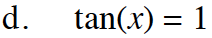### Home > INT3 > Chapter Ch12 > Lesson 12.1.1 > Problem12-5

12-5.
1. Decide if each of the following equations is always true, sometimes true, or never true. If an equation is always true, justify how you know; if it is sometimes true, give the exact values of x that make it true; and if it is never true, explain why it is never true. Homework Help ✎

1. 3x – 2 – 4(x + 1) = –x – 6

2. 3x – 5 = 2(x + 1) + x

3. sin(x) = cos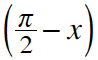4. tan(x) = 1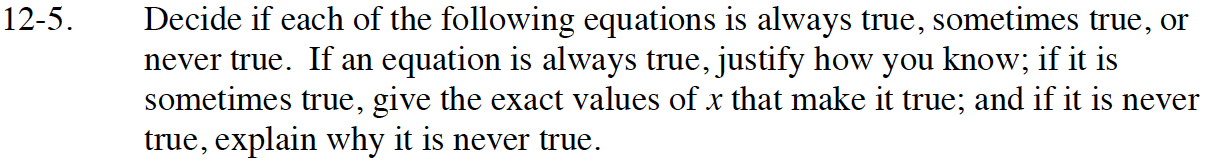Simplify the part of the equation on the left side of the equals sign.
Does it equal the part on the right side?

Always.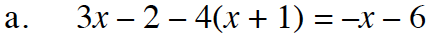Use the same method as part (a).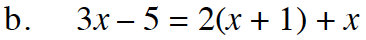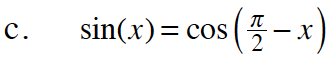Substitute values into the equations for x.
Are they always equal?

Always.

Is this always true?
If not, what values of x would make it true?